# SAT II Math I : Other 3-Dimensional Geometry

## Example Questions

### Example Question #1 : Other 3 Dimensional Geometry

A rectangular prism has a height of 4 in., length of 8in., and a width of 7in. Find the volume of the prism.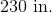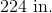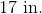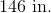Explanation:

To find the volume of a rectangular prism we use the equation of: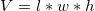Now substituting in our values we get: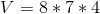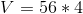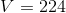### Example Question #2 : Other 3 Dimensional Geometry

What is the volume of a triangular prism having a base of 2, a height of 8, and second height of 14?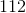Explanation:

To find the volume of a triangular prism we use the equation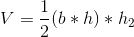In our case our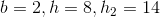Therefore,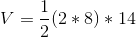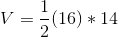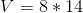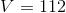### Example Question #3 : Other 3 Dimensional Geometry

If a cube has a surface area ofthen what is the the length of its longest diagonal?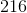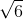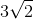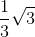Explanation:

The longest diagonal of a cube transverses the interior of the figure: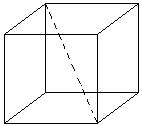This distance is defined by the super pythagorean theorem :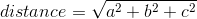where, andare the length, width, and height. Because the figure is a cube, all three are the same measure, and each is the side of the cube.

We can use the given surface area to find the length of the side: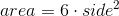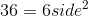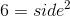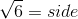We can use this value for the side to plug into the super pythagorean theorem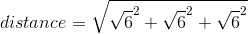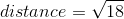Which can be simplified to